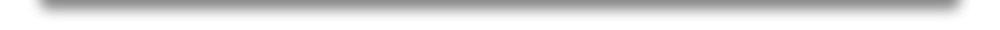## NodeBox for OpenGL » Geometry

NodeBox module nodebox.graphics.geometry is used in various other modules to help with mathematics. Many of its functions have been optimized for performance in C. NodeBox comes with precompiled C binaries for Mac OS X, but you can compile the C source code manually for other systems.

In the nodebox/ext/ folder, execute setup.py from the command line:

`> cd nodebox/ext/> python setup.py`

## Rotation

Trigonometry solves day-to-day tasks in computer graphics. The commands below can be used to find the distance and angle between points in 2D space. This is often very useful because the transformation state (i.e. the sequence of translate(), scale() and rotate() commands) do not give absolute coordinates; they work in a relative way.

When we know the angle of a shape we also know its "direction". Knowing the distance can tell us whether two shapes are going to intersect, or if they are close to each other and therefore in some way related. The reflection of a point can help us to construct smooth curves.

`angle(x0, y0, x1, y1)`
`distance(x0, y0, x1, y1)`
`coordinates(x0, y0, distance, angle)`
`rotate(x, y, x0, y0, angle)`
`reflect(x, y, x0, y0, d=1.0, a=180)`
• angle() returns the angle between points (x0,y0) and (x1,y1).
• distance() returns the distance between points (x0,y0) and (x1,y1).
• coordinates() returns the coordinates (x,y) of a point by rotating around origin (x0,y0).
• rotate() returns the coordinates of (x,y) rotated around origin (x0,y0).
• reflect() returns the reflection of a point (x,y) through origin (x0,y0).

## Interpolation

The lerp() command returns the linear interpolation between two numbers a and b, for time t between 0.0-1.0. For example: lerp(100, 200, t=0.5) means we get the number halfway between 100 and 200, which is 150.

The smoothstep() command returns a smooth transition between 0..0 and 1.0 using Hermite interpolation (cubic spline), where x is a number between a and b. The return value (0.0-1.0) will ease (slow down) as x nears a or b.

`lerp(a, b, t)                              # Returns a + (b-a)*t`
`smoothstep(a, b, x)                        # Returns 0.0-1.0 for a <= x <= b  `
`clamp(v, a, b)                             # Returns max(a, min(v, b)).`

## Intersection

The intersection() command determines if two lines – or a circle and a line – intersect. It returns a list of (x,y)-tuples, where each tuple is a point of intersection. For two lines, the list will be either empty or contain a single tuple. For a circle and a line, it can contain up to two tuples.

The point_in_polygon() command returns True when the given point (x,y) is inside a polygon (given as a list of (x,y)-tuples). A ray casting algorithm is used, which determines how many times a horizontal ray starting from the point intersects with the sides of the polygon. If it is an even number of times, the point is outside, if odd, inside.

`intersection(x1, y1, x2, y2, x3, y3, x4, y4, infinite=False)`
`intersection(x, y, radius, x1, y1, x2, y2, infinite=False)`
`point_in_polygon(points, x, y)`

References
: local.wasp.uwa.edu.au (1989), ecse.rpi.edu (1970)

## Transformation matrix

An m x n matrix is a table of numbers, with m rows and n columns. Because of the interesting calculations that are possible with and between matrices, they are used for many different purposes. An affine transformation matrix is a 3 x 3 matrix that represents position, scale, skew and rotation in a 2D space. Internally, it is used to draw transformed shapes to the canvas, or to find out if the mouse cursor is within a transformed layer.

Most notably, it can be used to transform the points of a BezierPath (there is a difference between drawing a path in the current transformation state or having an in-place transformed path).

`transform = AffineTransform(transform=None) `
`transform.copy()transform.prepend(transform)transform.append(transform)`
`transform.identitytransform.inversetransform.invert()transform.scale(x, y=None)transform.translate(x, y)transform.rotate(degrees=0, radians=0)`
`transform.transform_point(x, y)            # Returns a (x,y)-tuple.transform.transform_path(path)             # Returns a BezierPath.transform.map(points=[])                   # Returns a list of (x,y)-tuples.`
• The AffineTransform.transform_point() method applies the transformation matrix to a point and returns an (x,y)-tuple with the new coordinates.
• The AffineTransform.transform_path() method applies the transformation matrix to a BezierPath and returns a new BezierPath with updated coordinates.

References: senocular.com

## Point

The Point object can be used to represent a point in 2D space conveniently:

`pt = Point(x=0, y=0)`
`pt.xy                                      # Tuple of (x,y)-values.pt.x                                       # Horizontal position.pt.y                                       # Vertical position.`

## Bounds

The Bounds object can be used to represent a rectangular box that encompasses a transformed shape. It has a number of methods to find the encompassing bounds or overlapping parts between different bounds.

`b = Bounds(x, y, width, height)`
`b.xb.yb.widthb.height`
`b.copy()b.contains(x, y)                           # Returns True if (x,y) inside.b.contains(bounds)                         # Returns True if contains bounds.b.intersects(bounds)                       # Returns True if both bounds overlap.b.intersection(bounds)                     # Returns Bounds where both overlap.b.union(bounds)                            # Returns Bounds that encompass both.`

## Tessellation

OpenGL can only display simple convex polygons directly.
A polygon is simple if:

• the edges intersect only at vertices,
• there are no duplicate vertices,
• exactly two edges meet at any vertex.

Polygons containing holes or polygons with intersecting edges must first be subdivided into simple convex polygons before they can be displayed. Such subdivision is called tessellation.

The tessellate() command returns a list of triangulated (x,y)-vertices from the given list of path contours, where each contour is a list of (x,y)-tuples.

`tessellate(contours) `

The vertices can be drawn with GL_TRIANGLES to render a complex polygon, for example:

`from nodebox.graphics import geometryglBegin(GL_TRIANGLES)for x, y in geometry.tessellate(contours):    glVertex3f(x, y, 0)glEnd()`

The BezierPath object already does this for you.

References: supereffective.org (2008)